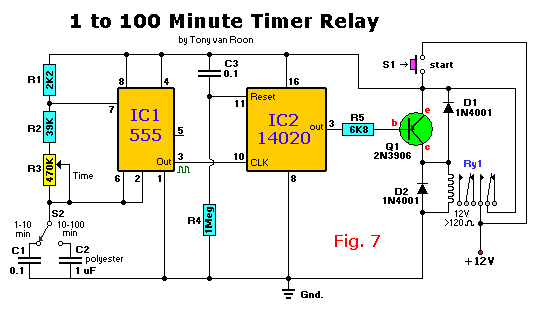```Parts List:
R1 = 2K2                 IC1 = 555, timer
R2 = 39K                 IC2 = 14020, (or 4020) divider
R3 = 470K (pot)           Q1 = 2N3906
R4 = 1M                D1,D2 = 1N4001
R5 = 6K8                  S1 = Push switch, momentary on
C1,C3 = 0.1uF, ceramic       S2 = Toggle switch, SPDT
C2 = 1uF, polyester      Ry1 = 12V
```
This is an accurate long-duration time delay driver, switchable between 1 and 10 minutes or 10 to 100 minutes and whose function does not depend on electrolytic capacitors. Film dielectric caps have been selected. IC1 is configured as a free-running astable multivibrator which frequency is divided down by IC2, a 14020 (or 4020) CMOS 14-stage, ripple-carry binary divider. Consequently relay RY1 turns on as soon as switch S1 is closed, and it turns off again when the 8192nd astable pulse arrives. This method provides time periods ranging from 1 to 100 minutes, depending on the switch setting of S2.